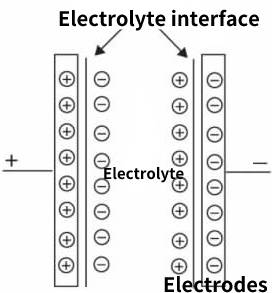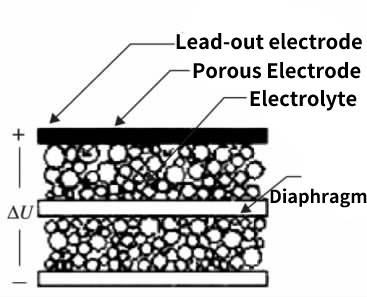Supercapacitors, also known as ultracapacitors or electrochemical capacitors, are emerging as a promising energy storage technology for a variety of applications. Unlike conventional batteries, which store energy through chemical reactions, supercapacitors pacitors store energy electrostatically, enabling them to charge and discharge much faster and more efficiently. In this article, we’ll explore the working principle of supercapacitor.

## 1.1 Working principle of supercapacitor-electric double layer

As early as 1879, the German Heimholtz (Heimholtz) discovered the principle of the electric double layer, which is the working principle of supercapacitor  (also known as the electric double layer capacitor). The working principle of the electric double layer capacitor is based on the basic principle of electrochemistry, after the contact between the conductor and the electrolyte (liquid and solid), the surface (that is, the interface) generates a stable double-layer charge (electric double layer) with opposite signs. Under a certain voltage, the charge cannot be pulled by the electric field generated by the electric double layer charge to the electrode close to it and opposite in sign, forming two electrodes of the actual capacitor, as shown in Figure 6.1. The electric charge of the electrolyte in the electric double layer electric charge appears in the form of ions, and its size is only about nanometers, so that the distance between the two electrodes of this de facto capacitor is only nanometers, thus obtaining a nanometer-scale electrode distance .Figure 6-1 Principle of electric double layer

## 1.2 Obtaining the supercapacitance of supercapacitorsFigure 6-2  Supercapacitor structure

It can be seen from the figure 6.2 that the actual electrode of the supercapacitor is an activated carbon porous electrode, and the lead-out electrode is aluminum foil, which is similar to the cathode of an aluminum electrolytic capacitor; the electrolytic capacitor paper is used as the diaphragm of the two electrodes in the middle, except for the lead-out All space outside the activated carbon and separator is filled with electrolyte, which allows a much larger area than the actual area-to-space ratio of deeply etched aluminum foil. Similarly, the fluid electrolyte can be in close contact with the porous activated carbon electrode, so that the actual electrode has a larger effective plate area, which can reach 200 square meters per gram.

The capacitance of the plate capacitor is

C=Ɛo·Ɛ·S/d

Among them, Ɛo, Ɛ, S, and d are the dielectric coefficient of the relative vacuum of the medium between the plates, the relative dielectric constant of the dielectric, the plate area of the capacitor, and the distance between the plates.

Although the actual electrode of the supercapacitor is activated carbon powder, it is still much larger than the ionic half of the electrolyte, so the capacity formula of the plate capacitor can still be applied.

To sum up, the combination of the structure in Figure 6.2 and the principle of the electric double layer forms a supercapacitor with a very large capacitance, even if the capacitance is thousands of farads, it is not surprising.

## 1.3 Electrodes of the earliest supercapacitors

In order to obtain the porous electrode of the supercapacitor, the electrode was initially made of gold! Therefore, supercapacitors are also called “gold capacitors” in many places. To this day, Matsushita Electric of Japan calls its supercapacitors “golden capacitors.” Due to the use of expensive gold as the electrode, the price of supercapacitors must be expensive, and it is impossible to get practical applications.

If this kind of madness can bring about the energy storage and extremely fast discharge capability of capacitors, the short-term and extremely high-power power requirements for laser weapons can be realized. As a military use, it is still valuable. The price of an American F22 fighter jet The best explanation is that it is more expensive than gold of the same weight. Judging from the performance-price ratio of “golden capacitors”, the price is much higher than the use value, which is the fundamental reason why “golden capacitors” have withdrawn from the stage of history.

## 1.4 Difference between supercapacitor and electrolytic capacitor

On the surface, both supercapacitors and electrolytic capacitors have electrolyte, so the characteristics of supercapacitors will also be similar to those of electrolytic capacitors, and supercapacitors with pure electric double layer principles, like electrolytic capacitors, do not allow electrochemical processes. The working principle of supercapacitor is fundamentally different from that of electrolytic capacitors. The electrolyte in an electrolytic capacitor is the actual cathode, while the electrolyte in a supercapacitor is the middle “electrode”, the actual electrode is porous activated carbon, and the medium is the “space” between the electrolyte and the actual electrode. medium. Common electrolytic capacitors use threshold metals as electrodes and their oxides as dielectrics. Therefore, electrolytic capacitors are polarized and reverse voltage is not allowed. As can be seen from Figure 6.1, the two electrode structures of the supercapacitor have the same form, so the supercapacitor is a non-polar capacitor, which can apply both “positive” and “reverse” voltages. This is where supercapacitors differ from electrolytic capacitors.

The supercapacitor is the same as the electrolytic capacitor in that both increase the effective area of the electrodes as much as possible and reduce the distance between the electrodes as much as possible, so as to obtain high capacitance. Tantalum electrolytic capacitors use the same method of increasing the effective electrode area as supercapacitors.